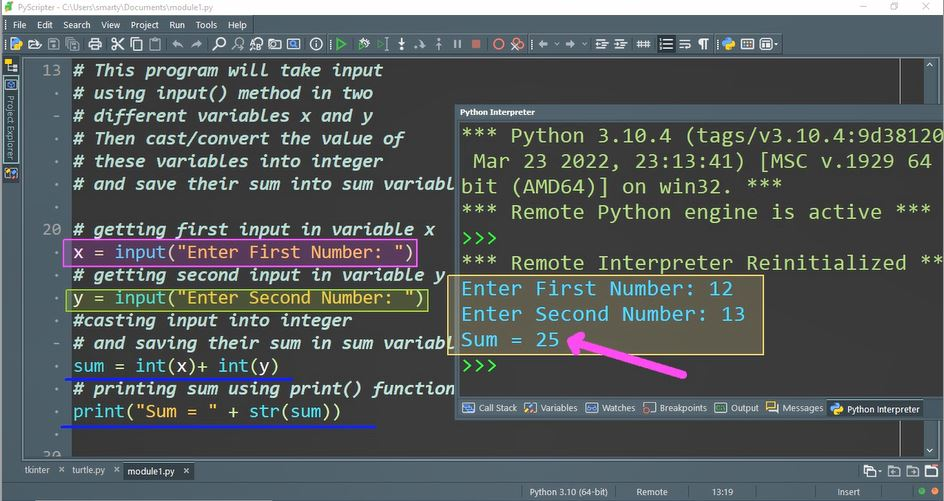# Python Program To Add Two Numbers using user input

## Source Code:

# This program will take input
# using input() method in two
# different variables x and y
# Then cast/convert the value of
# these variables into integer
# and save their sum into sum variable

# getting first input in variable x
x = input("Enter First Number: ")
# getting second input in variable y
y = input("Enter Second Number: ")
#casting input into integer
# and saving their sum in sum variable
sum = int(x) + int(y)
# printing sum using print() function
print("Sum = " + str(sum))

## Output:## Working:

In this program example two variables are used for storing user input named as x and y. After taking input the value and types of both variables are converted using conversion int() method.

In python variables are not declared along with specific data type. The type of variable depends on data it will store. So that sum variable data type will be integer automatically.

In print statement, that will show sum of this input we are concatenating constant string   "Sum = "  with sum variable.

According to syntax/rules we cannot concatenate string constant with any integer variable. So that again in print() function we are using str() string cast function. This will convert type of sum variable into string. And perform its concatenation with string constant.